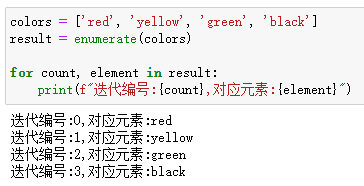# Python中號稱神仙的六個內置函數詳解## Lambda 函數

Lambda函數用於創建匿名函數，即沒有名稱的函數。它隻是一個表達式，函數體比def簡單很多。當我們需要創建一個函數來執行單個操作並且可以在一行中編寫時，就可以用到匿名函數瞭。

```lambda [arg1 [,arg2,.....argn]]:expression
```

lambda的主體是一個表達式，而不是一個代碼塊。僅僅能在lambda表達式中封裝有限的邏輯進去。例如：

`lambda x: x+2`

```add2 = lambda x: x+2
```## Map 函數

map()函數會將一個函數映射到一個輸入列表的所有元素上。

`map(function,iterable)`

```def makeupper(word):
return word.upper()
colors=['red','yellow','green','black']
colors_uppercase=list(map(makeupper,colors))
colors_uppercase``````colors=['red','yellow','green','black']
colors_uppercase=list(map(lambda x: x.upper(),colors))
colors_uppercase```## Reduce函數

```reduce(function, iterable[, initializer])
```

```from functools import reduce
def add(x, y) :   # 兩數相加
return x + y
numbers = [1,2,3,4,5]
sum1 = reduce(add, numbers)   # 計算列表和
```▲代碼執行過程動圖

```from functools import reduce
numbers = [1,2,3,4,5]
sum2 = reduce(lambda x, y: x+y, numbers)```

## enumerate 函數

enumerate()函數用於將一個可遍歷的數據對象(如列表、元組或字符串)組合為一個索引序列，同時列出數據和數據下標，一般用在for循環當中。它的語法如下所示：

`enumerate(iterable, start=0)`

```colors = ['red', 'yellow', 'green', 'black']
result = enumerate(colors)```

```for count, element in result:
print(f"迭代編號:{count},對應元素:{element}")```## Zip 函數

zip()函數用於將可迭代的對象作為參數，將對象中對應的元素打包成一個個元組，然後返回由這些元組組成的列表。

```colors = ['red', 'yellow', 'green', 'black']
fruits = ['apple', 'pineapple', 'grapes', 'cherry']
for item in zip(colors,fruits):
print(item)``````prices =[100,50,120]
for item in zip(colors,fruits,prices):
print(item)```## Filter 函數

filter()函數用於過濾序列，過濾掉不符合條件的元素，返回由符合條件元素組成的新列表，其語法如下所示。

`filter(function, iterable)`

```def is_odd(n):
return n % 2 == 1
old_list = [1, 2, 3, 4, 5, 6, 7, 8, 9, 10]
new_list = filter(is_odd, old_list)
print(newlist)```